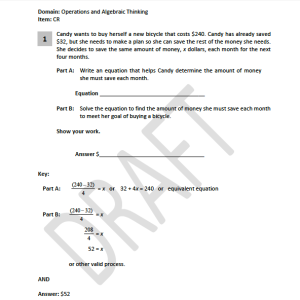# NY students, it’s not you, it’s them

June 8, 2013

How do Common Core (CC) test makers think a fourth grader would be able to solve this problem in the way the answer key provides? For example, they expect the student to write an equation: 32+4x=240. Really? IF, a child could write this equation from reading the problem after fourth grade CC textbooks, I would be surprised.  A multi-step problem written as one equation, has not been taught. Also, have they  learned about adding variables with a coefficient? No. If they wrote this equation, which I doubt many would be able to,  they very likely would add 32+4x=36X. They haven’t learned the fundamentals of algebra to make sense of this. Let alone how to isolate x and keep the equation balanced.

Secondly, They aren’t teaching the standard algorithm for division until sixth grade with CC. Do they expect the kids to draw 208 items, then group them into sets of 4? Do they expect them to substitute several numbers into THE multiplication of 4x?=208. That takes some time, 4×20=80, no, 4×30=120, no,it could go on and on.

OR even better, the CC teaches to break down 208 into 200+8, hmm, then the kid says, “I know 4X50=200 AND 2X4=8 SO, 200/4=5 AND 8/4=2, THEREFORE I CAN ADD THE DIVIDENDS TOGETHER AND GET 52. YEAH, THAT’S IT, I’M A MATHEMATICIAN.” Check out the learning channel’s CC lesson on division https://www.teachingchannel.org/videos/common-core-teaching-division, it’s a great one.

The problem’s answer key for the test does not reflect any of these methods, no wonder the kids in NY did so poorly.

This problem would not be difficult for child in Japan, because they are taught the standard algorithms, for all operations, before they are expected to use them in algebraic problems solving of this type. CC wants kids to think like mathematicians, only they don’t give them the tools necessary to do so. Click on image for larger view.Filed in: Uncategorized